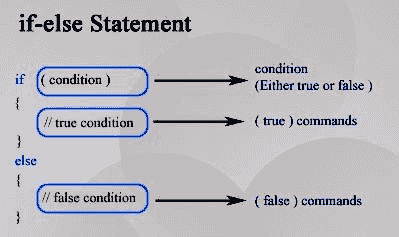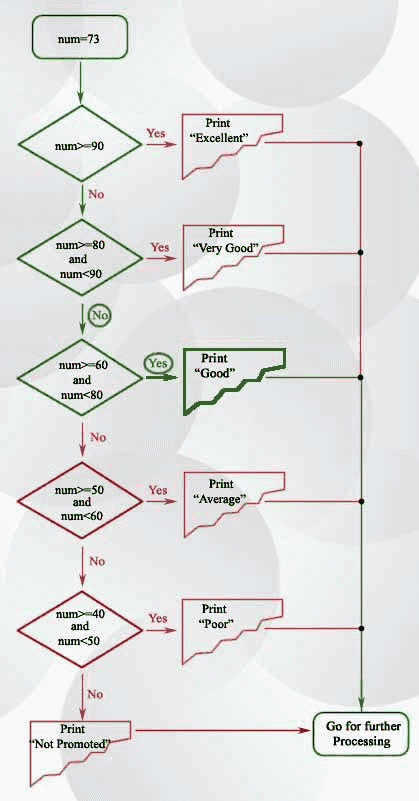# C if else

## Introduction

The if statement is used to conditionally execute a statement or a block of statements. Conditions can be true or false, execute one thing when the condition is true, something else when the condition is false.

Syntax:

```if (expression)
statement(s);
[else statement(s);]
```

The expression after the 'if' is called the condition of the if statement. If the expression is evaluated to true, the statement(s) following 'if' clause execute and if the expression evaluates to a false value, the statement following the 'else' clause execute. See the following pictorial presentation and a simple example of if-else statement.Example:

``````if (a == b)
printf ("%d is equal to %d", a, b);
else
printf ("%d is not equal to %d", a, b);
``````

## If-then statements:

If we want to execute some statement(s) under a given condition and nothing otherwise then the syntax will be :

```  if (expression)

statement(s);  ```

Example:

``````if (a == b)
printf ("if a is equal to b");
printf ("%d is equal to %d", a, b);
``````

Note : There is no indentation rule in writing C programming, we can write the above code in following ways :

``````if (a == b)
printf ("if a is equal to b");
printf ("%d is equal to %d", a, b);
``````
``````if (a == b)
{
printf ("if a is equal to b");
printf ("%d is equal to %d", a, b);
}
``````

The second way of writing code is a good practice.

## A complete example of conditional if-else statement

The following program takes an integer (an integer is a number that can be written without a fractional or decimal component) as input from the user and finds whether the given number is positive or negative.

``````#include<stdio.h>
main()
{
int num;
printf("Input a number : ");
scanf("%d",&num);
if(num>0)
{
printf("This is a positive integer\n");
}
else // else portion of if statement
{
printf(" This is not a positive integer..Try again\n");
}
}
``````

The following program works same as that of the above program. But here we use two separate if-statements.

``````#include<stdio.h>
main()
{
int num;
printf("Input an integer : ");
scanf("%d",&num);
if(num>0)
{
printf("This is a positive integer\n");
}
if(num>=0)
{
printf(" This is not a positive integer..Try again\n");
}
}``````

## Sequential if-then statements

You can use sequential if-statements when conditions are not mutually exclusive. See the following example where we want to print messages to tell which of three variables (between a, b, c) are the same :

``````if (a == b)
printf ("a = b");
if (a == c)
printf ("a = c");
if (b == c)
printf ("b = c")``````

## Multiway if-else-Statement

The syntax of the multi-way if-else-statement is :

``````if (expression_1)
statement_1
else if (expression_2)
statement_2
.
.
.
else if (expression_n)
statement_n
else
other_statement
``````

At the time of execution the compiler will check the expression (condition) one after the other, if any expression (condition) is found to hold true, the corresponding statement will be executed. If none of the expression (condition) is true then the statement after the last else will be executed.

Example:

For example, there is a grading system in an examination evaluation. The rules are defined in the following table.

>=90% Excellent
Between 80% and 89% Very Good
Between 60% and 79% Good
Between 50% and 59% Average
Between 40% and 49% Poor
<40% Not promoted

The following program determines the grade of a student according to the rules of the said table. The program needs to input score of a student.

``````#include<stdio.h>
main()
{
int num;
printf("Input score :");
scanf("%d",&num);
if (num>=90)
{
}
else if(num>=80 && num<90)
{
}
else if(num>=60 && num<80)
{
}
else if(num>=50 && num<60)
{
}
else if(num>=40 && num<50)
{
}
else
{
}
}
``````

Here is the flowchart of the said program :## Nested if-then-else statements

Each if-statement is itself a statement, so it can be nested inside another if statement. Nesting can go on indefinitely. See the following example (nested if-else is used), which find minimum among three numbers.

``````#include<stdio.h>
main()
{
int num1=5, num2=3, num3=-12, min;
if(num1<num2)
{
if(num1<num3)
min = num1;
else
min = num3;
}
else
{
if(num2<num3)
min = num2;
else
min = num3;
}
printf("Among %d, %d, %d minimum number is %d",num1,num2,num3,min);
}
``````

Output:

`Among 5, 3, -12 minimum number is -12`

Previous: C printf()
Next: C for loop

﻿

## C Programming: Tips of the Day

What does [ N ... M ] mean in C aggregate initializers?

It is initialization using Designated Initializers.

The range based initialization is a gnu gcc extension.

To initialize a range of elements to the same value, write [first ... last] = value. This is a GNU extension. For example,

``` int widths[] = { [0 ... 9] = 1, [10 ... 99] = 2,  = 3 };
```

It is not portable. Compiling with -pedantic with tell you so.

How does it work here?

The preprocessor replaces #include <asm/unistd.h> with its actual contents(it defines miscellaneous symbolic constants and types, and declares miscellaneous functions) in the range based construct, which are then further used for initializing the array of pointers.

Ref : https://bit.ly/2SQE4wm